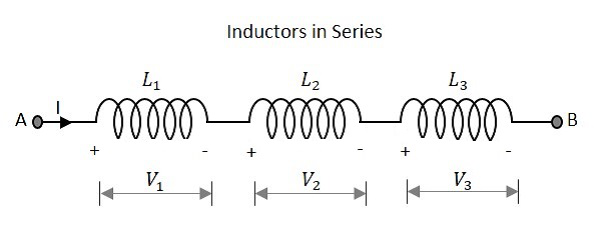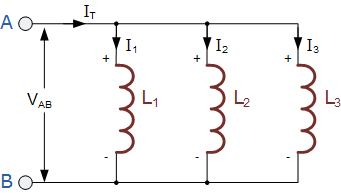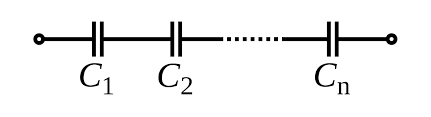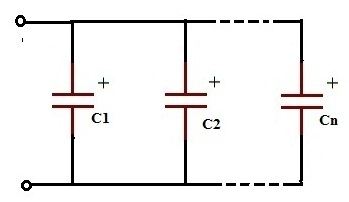### Inductor in Series

Inductor in Series:
Series connection of the inductor is similar to the resistor.V = V1 + V2 +V3

Leq = L1 + L2 + L3

V ∝ L

Inductors in Parallel:

Parallel connection of the inductor is similar to the resistor.I = I1 + I2 + I3
I ∝ 1/L
1/Leq = 1/L1 + 1/L2 + 1/L3

Capacitor in Series:

In the case of capacitors, series combination represents a parallel combination of resistance.Capacitors in parallel:

Parallel combination of capacitors represent series combination of resistor.Ceq = C1 + C2 + ……. + Cn

Note

• Always first solve the problem using a series and parallel method.

• In series resistance, the equivalent resistance is maximum of maximum.

• In parallel resistance, the equivalent resistance is minimum of minimum